Ask your WordPress questions! Pay money and get answers fast!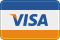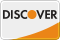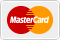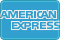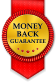# Custom Function Stopped Working WordPress

• SOLVED

We created this custom function to basically allow for a user to submit a post and give it a date. The post was supposed to go live on that date and then automatically be pulled down on the end date or 14 days later (whichever came first). It did so by checking hourly to see if the date criteria fit and changing the post from "Pending" to "Published" and vice versa. For some reason this functionality is no longer working and posts are not going live when they should and some are not coming down when they should. I'm not sure if there is a small error in the code or if this is a bigger issue so I'm putting the prize at \$30 and if its a bigger issue I will pay more.

```// converts a time stamp to date string for meta fields if(!function_exists('ecpt_timestamp_to_date')) { function ecpt_timestamp_to_date(\$date) { return date('m/d/Y', \$date); } } if(!function_exists('ecpt_format_date')) { function ecpt_format_date(\$date) { \$date = strtotime(\$date); return \$date; } } add_filter("gform_post_data", "set_post_date", 10, 3); function set_post_date(\$post_data, \$form, \$entry){ //only do this when the form id is 23 if(\$form["id"] != 5) return \$post_data; //set post date to field on form if date provided; get from entry data //using a date field in mm/dd/yyyy format and a time field in 24 hour format \$date_value = \$entry["11"]; //pull the date value from the form data posted, field 6 on my form \$time = \$entry[""]; //pull the time value from the form data posted, field 7 on my form //only change post date/time when a date has been chosen if (!empty(\$date_value)) { if (empty(\$time)) { //set default time \$time_value = "00:00:00"; } else { empty(\$time) ? \$time_hours = "00" : \$time_hours = \$time; //pull hours out of array empty(\$time) ? \$time_minutes = "00" : \$time_minutes = \$time; //pull minutes out of array \$time_value = \$time_hours . ":" . \$time_minutes . ":00"; //add on seconds } //convert post date to the appropriate format so it will be inserted into the database \$post_date = date("Y-m-d H:i:s",strtotime(\$date_value . " " . \$time_value)); \$post_data["post_date"] = \$post_date; //set the post_date } return \$post_data; //return the changed data to use when the post is created } // create cron that will check for correct published post each day if (!wp_next_scheduled('g_post_status')) { wp_schedule_event( time(), 'hourly', 'g_post_status' ); } // uncomment below to clear this cron // wp_clear_scheduled_hook('g_post_status'); // perform search for post at certain date, and change post to publish for correct time condition function expire_submitted_posts(){ // custom query argument \$publish_args = array( 'category_name' => 'free-ebooks', 'posts_per_page' => -1 ); \$pending_args = array( 'category_name' => 'free-ebooks', 'posts_per_page' => -1, 'post_status' => 'pending' ); \$meta_start_date_key = 'download_start_date'; \$meta_end_date_key = 'download_end_date'; \$current_time = time(); \$published_posts = get_posts(\$publish_args); \$pending_posts = get_posts(\$pending_args); foreach(\$published_posts as \$post){ \$this_post = array(); \$this_post['ID'] = \$post->ID; // get start and end time from custom fields \$start_publish_date = strtotime(get_post_meta(\$post->ID, \$meta_start_date_key, true)); \$end_publish_date = strtotime(get_post_meta(\$post->ID, \$meta_end_date_key, true)); // set maximum 14 days from start time \$max_end_time = \$start_publish_date + (14 * 24 * 60 * 60); // we update post to draft if more than 14 days form start or more than end date if( \$current_time > \$max_end_time || \$current_time > \$end_publish_date){ \$this_post['post_status'] = 'draft'; wp_update_post( \$this_post ); } } foreach(\$pending_posts as \$post){ \$this_post = array(); \$this_post['ID'] = \$post->ID; // get start and end time from custom fields \$start_publish_date = strtotime(get_post_meta(\$post->ID, \$meta_start_date_key, true)); \$end_publish_date = strtotime(get_post_meta(\$post->ID, \$meta_end_date_key, true)); if( \$start_publish_date < \$current_time && \$current_time < \$end_publish_date){ \$this_post['post_status'] = 'publish'; wp_update_post( \$this_post ); } } } add_action( 'g_post_status', 'expire_submitted_posts' ); ```

2014-01-15

is it creating posts or not?

Its creating the posts but they aren't triggering on and off like they are supposed to. Some of them are but a lot of them aren't

can you provide me access to your site , I would like to check it there , my email is : [email protected]

i need ftp and admin access.

Ok, I'll set you up with a temp login

2014-01-15